# Example Applications

This page provides a list of libROM example applications. For detailed documentation of the libROM sources, including the examples, see the online Doxygen documentation or the doc directory in the distribution. The goal of the example codes is to provide a step-by-step introduction to libROM in simple model settings.

Select from the categories below to display examples and miniapps that contain the respective feature. All examples support (arbitrarily) high-order meshes and finite element spaces. The numerical results from the example codes can be visualized using the GLVis or VisIt visualization tools. See the GLVis and VisIt websites for more details.

Users are encouraged to submit any example codes and miniapps that they have created and would like to share.
Contact a member of the libROM team to report bugs or post questions or comments.

## Global pROM for Poisson problem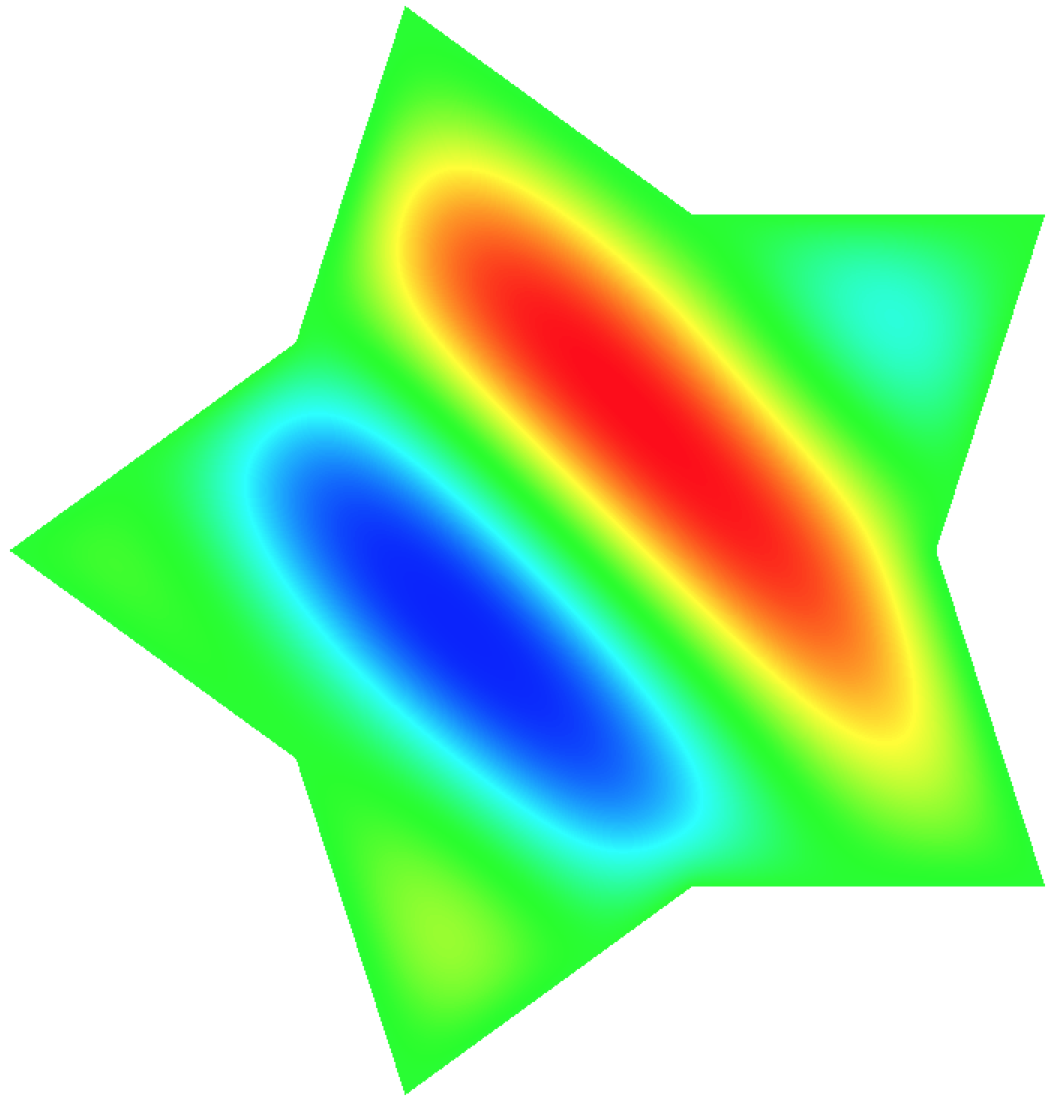This example code demonstrates the use of libROM and MFEM to define a reduced order model for a simple isoparametric finite element discretization of the Poisson problem $$-\Delta u = f$$ with homogeneous Dirichlet boundary conditions. The related tutorial YouTube video can be found here. The example parameterizes the righthand side with frequency variable, $\kappa$:

$$f = \cases{ \displaystyle \sin(\kappa (x_0+x_1+x_2)) & for 3D \cr \displaystyle \sin(\kappa (x_0+x_1)) & for 2D }$$

The 2D solution contour plot for $\kappa=\pi$ is shown in the figure on the right to show the effect of $\kappa$. For demonstration, we sample solutions at $\kappa=\pi$, $1.1\pi$, and $1.2\pi$. Then a ROM is build with basis size of 3, which is used to predict the solution for $\kappa = 1.15\pi$. The ROM is able to achieve a speedup of $7.5$ with a relative error of $6.4\times10^{-4}$. One can follow the command line options below to reproduce the numerical results summarized in the table below:

• offline1: poisson_global_rom -offline -f 1.0 -id 0
• offline2: poisson_global_rom -offline -f 1.1 -id 1
• offline3: poisson_global_rom -offline -f 1.2 -id 2
• merge: poisson_global_rom -merge -ns 3
• reference FOM solution: poisson_global_rom -fom -f 1.15
• online: poisson_global_rom -online -f 1.15

The command line option -f defines a frequency of the sinusoidal right hand side function. The relation between $kappa$ and f is defined as $\kappa = \pi f$.

FOM solution time ROM solution time Speed-up Solution relative error
0.22 sec 0.029 sec 7.5 6.4e-4

The code that generates the numerical results above can be found in (poisson_global_rom.cpp) and the explanation of codes is provided in here. The poisson_global_rom.cpp is based on ex1p.cpp from MFEM with a modification on the right hand side function.

## Greedy pROM for Poisson problem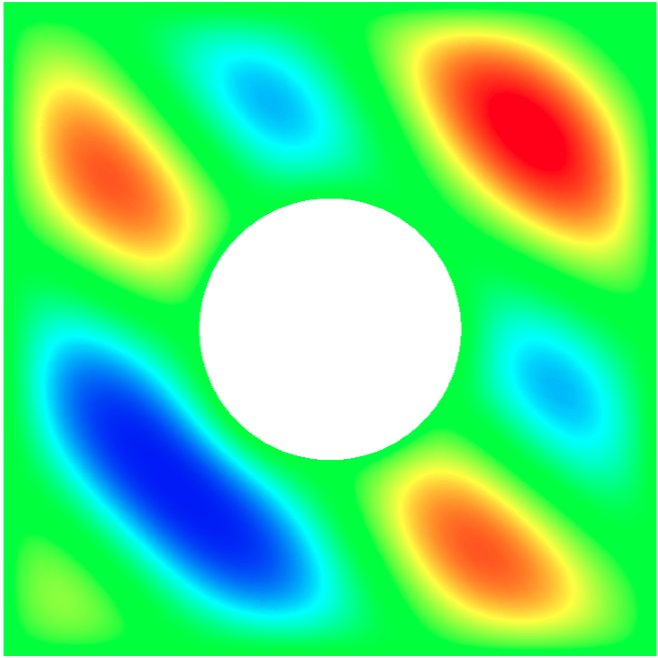This example code demonstrates physics-informed greedy sampling procedure of building local pROMs for the Poisson problem. $$-\Delta u = f$$ with homogeneous Dirichlet boundary conditions.
The example parameterizes the righthand side with frequency variable, $\kappa$:

$$f = \cases{ \displaystyle \sin(\kappa (x_0+x_1+x_2)) & for 3D \cr \displaystyle \sin(\kappa (x_0+x_1)) & for 2D }$$

A set of local ROMs are built for chosen parameter sample points. The parameter sample points are chosen through physics-informed greedy procedure, which is explained in detail by the tutorial YouTube video. Then the local ROMs are interpolated to build a tailored local ROM for a predictive case. Unlike the global ROM, the interpolated ROM has dimension that is the same as the individual local ROM.

For example, one can follow the command line options below to reproduce the numerical results summarized in the table below:

• greedy step: poisson_local_rom_greedy -build_database -greedy-param-min 0.5 -greedy-param-max 3.0 -greedy-param-size 15 -greedysubsize 4 -greedyconvsize 6 -greedyrelerrortol 0.01 --mesh "../../../dependencies/mfem/data/square-disc-nurbs.mesh"

This particular greedy step generates local pROMs at the following 8 parameter points, i.e., 0.521923, 0.743108, 1.322449, 1.754950, 2.011140, 2.281129, 2.587821, 2.950198.

• reference FOM solution: poisson_local_rom_greedy -fom --mesh "../../../dependencies/mfem/data/square-disc-nurbs.mesh" -f X.XX
• online: poisson_local_rom_greedy -use_database -online --mesh "../../../dependencies/mfem/data/square-disc-nurbs.mesh" -f X.XX

You can replace X.XX with any value between 0.5 and 3.0. The table below shows the performance results for three different parameter points.

X.XX FOM solution time ROM solution time Speed-up Solution relative error
1.0 0.0135 sec 2.38e-6 sec 5.7e3 9.99593e-5
2.4 0.0137 sec 2.48e-6 sec 5.5e3 0.0001269
2.8 0.0159 sec 2.92e-6 sec 5.4e3 0.00126

The code that generates the numerical results above can be found in (poisson_local_rom_greedy.cpp). The poisson_local_rom_greedy.cpp is based on ex1p.cpp from MFEM with a modification on the right hand side function.

## DMD for heat conductionFor a given initial condition, i.e., $u_0(x) = u(0,x)$, heat conduction solves a simple 2D/3D time dependent nonlinear heat conduction problem

$$\frac{\partial u}{\partial t} = \nabla\cdot (\kappa + \alpha u)\nabla u,$$

with a natural insulating boundary condition $\frac{du}{dn}=0$. We linearize the problem by using the temperature field $u$ from the previous time step to compute the conductivity coefficient.

One can run the following command line options to reproduce the DMD results summarized in the table below:

• heat_conduction -s 3 -a 0.5 -k 0.5 -o 4 -tf 0.7 -vs 1 -visit
FOM solution time DMD setup time DMD query time DMD relative error
4.8 sec 0.34 sec 1.4e-3 sec 8.2e-4

The code that generates the numerical results above can be found in (heat_conduction.cpp). The heat_conduction.cpp is based on ex16p.cpp from MFEM.

## Parametric DMD for heat conduction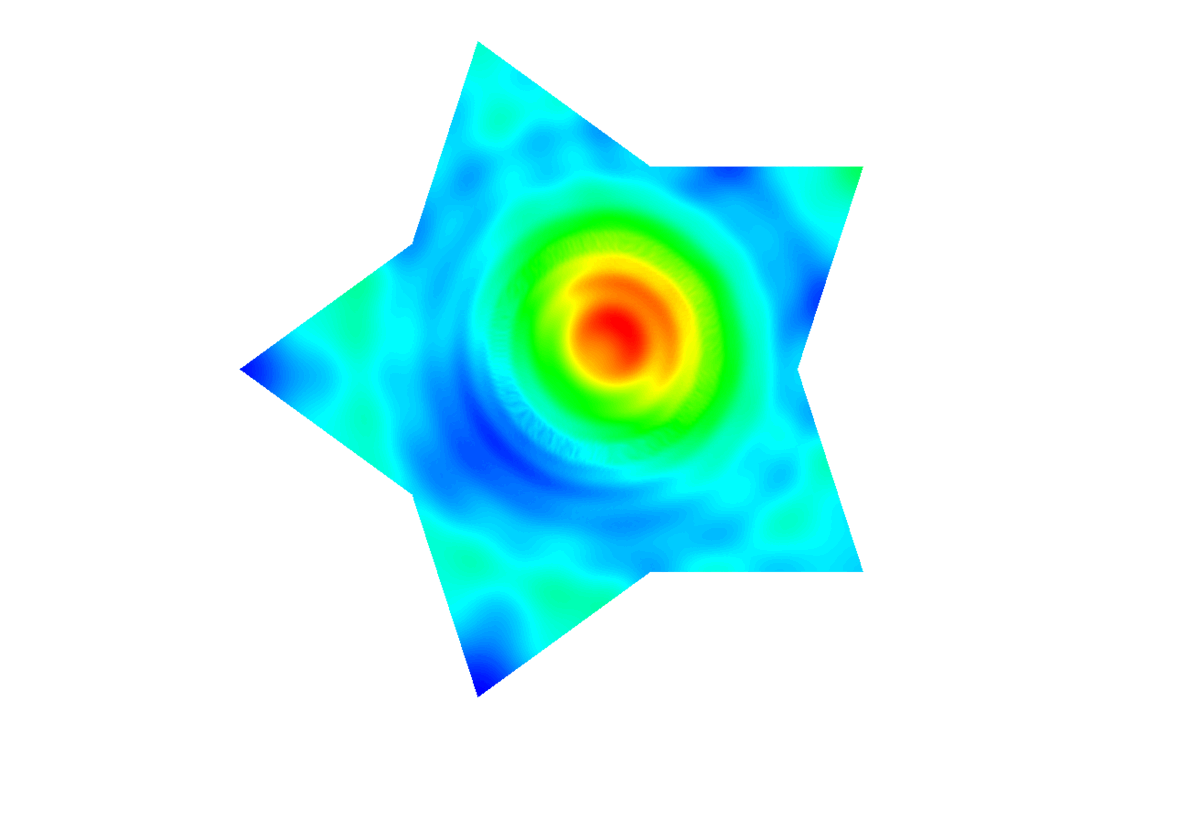This example demonstrates the parametric DMD on the heat conduction problem. The initial condition, $u_0(x)$, is parameterized by the center of circle and the radius, i.e.,

$$u_0(x) = \cases{ \displaystyle 2 & for |x-c| < r \cr \displaystyle 1 & for |x-c| \ge r }$$

One can run the following command line options to reproduce the parametric DMD results summarized in the table below:

• rm -rf parameters.txt
• parametric_heat_conduction -r 0.1 -cx 0.1 -cy 0.1 -o 4 -visit -offline -rdim 16
• parametric_heat_conduction -r 0.1 -cx 0.1 -cy 0.5 -o 4 -visit -offline -rdim 16
• parametric_heat_conduction -r 0.1 -cx 0.5 -cy 0.1 -o 4 -visit -offline -rdim 16
• parametric_heat_conduction -r 0.1 -cx 0.5 -cy 0.5 -o 4 -visit -offline -rdim 16
• parametric_heat_conduction -r 0.5 -cx 0.1 -cy 0.1 -o 4 -visit -offline -rdim 16
• parametric_heat_conduction -r 0.25 -cx 0.2 -cy 0.4 -o 4 -visit -online -predict
• parametric_heat_conduction -r 0.4 -cx 0.2 -cy 0.3 -o 4 -visit -online -predict

where r, cx, and cy specify the radius, the x and y coordinates of circular initial conditions.

r cx cy FOM solution time DMD setup time DMD query time DMD relative error
0.25 0.2 0.4 13.3 sec 0.34 sec 1.2 sec 7.0e-3
0.2 0.4 0.2 13.8 sec 0.32 sec 1.2 sec 3.9e-3
0.3 0.3 0.3 13.6 sec 0.33 sec 1.1 sec 1.3e-2
0.3 0.4 0.2 14.1 sec 0.34 sec 1.3 sec 8.4e-3
0.2 0.3 0.4 14.2 sec 0.34 sec 1.3 sec 7.9e-3
0.4 0.2 0.3 13.9 sec 0.36 sec 1.5 sec 9.0e-3

The code that generates the numerical results above can be found in (parametric_heat_conduction.cpp). The parametric_heat_conduction.cpp is based on ex16p.cpp from MFEM.

## Optimal control for heat conduction with DMD and differential evolution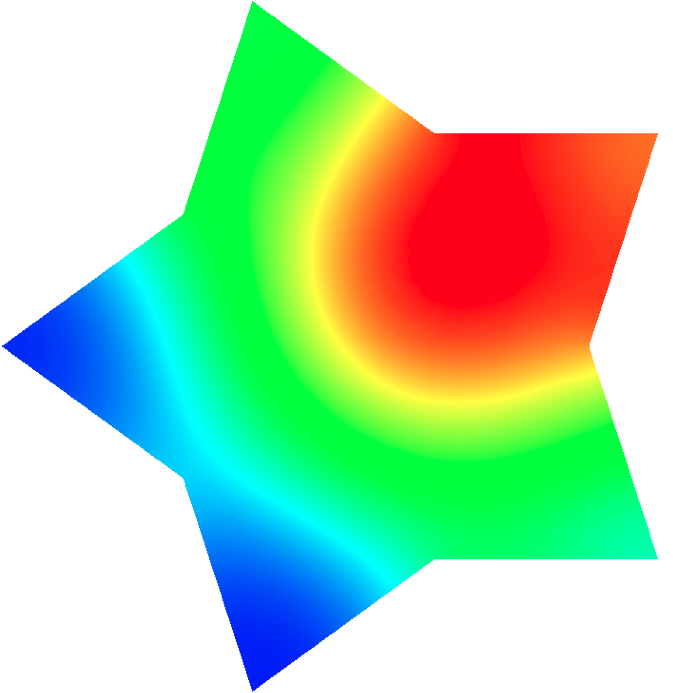This example demonstrates the optimal control heat conduction problem with greedy parametric DMD and differential evolution. The initial condition, $u_0(x)$, is parameterized by the center of circle and the radius, i.e.,

$$u_0(x) = \cases{ \displaystyle 2 & for |x-c| < r \cr \displaystyle 1 & for |x-c| \ge r }$$

The goal of the optimal control problem is to find an initial condition that achieves the target last time step temperature distribution. If it does not achieve the target, then it should be closest, given the initial condition parameterization. It is formulated mathematically as an optimization problem:

$$\underset{c,r}{minimize} \ || u_T(c,r) - u_{target} ||_2^2,$$

where $u_T$ denotes the last time step temperature and $u_{target}$ denotes the target temperature. Note that $u_T$ depends on the initial condition parameters, i.e., $c$ and $r$. It means that we obtain $u_T$ by solving a forward heat conduction problem. As you can imagine, it needs to explore the parameter space and try to find $c$ and $r$ that produces $u_T$ that best matches $u_{target}$. If each solution process of heat conduction problem is computationally expensive, the search for the optimal parameter can take a while. Therefore, we use our parametric DMD to expedite the process and the search algorithm is done by the differential evolution.

Here are the steps to solve the optimal control problem. First, you must delete any post-processed files from the previous differential evolution run. For example,

• rm -rf parameters.txt
• rm -rf de_parametric_heat_conduction_greedy_*

Then create parametric DMD using a greedy approach with physics-informed error indicator:

• de_parametric_heat_conduction_greedy -build_database -rdim 16 -greedy-param-size 20 -greedysubsize 10 -greedyconvsize 15 -greedyreldifftol 0.0001

Then you can generate target temperature field with a specific $r$ and $c$ values. Here we used $r=0.2$, $cx=0.2$, and $cy=0.2$ to generate a target temperature field. The target temperature field is shown in the picture above (the one on the left).

Therefore, if DMD is good enough, the differential evolution should be able to find $c$ and $r$ values that are closed to these:

• de_parametric_heat_conduction_greedy -r 0.2 -cx 0.2 -cy 0.2 -visit (Compute target FOM)

where r, cx, and cy specify the radius, the x and y coordinates of circular initial conditions. Now you can run the differential evolution using the parametric DMD:

• de_parametric_heat_conduction_greedy -r 0.2 -cx 0.2 -cy 0.2 -visit -de -de_f 0.9 -de_cr 0.9 -de_ps 50 -de_min_iter 10 -de_max_iter 100 -de_ct 0.001 (Run interpolative differential evolution to see if target FOM can be matched)

The differential evolution should be able to find the following optimal control parameters, e.g., in Quartz: $r=0.2002090156652667$, $cx=0.2000936529076073$, and $cy=0.2316380936755735$, which are close to the true parameters that were used to generate the targer temperature field. The DMD temperature field at the last time step on this control parameters is shown in the picture above (the one on the right).

The code that generates the numerical results above can be found in (de_parametric_heat_conduction_greedy.cpp). The de_parametric_heat_conduction_greedy.cpp is based on ex16p.cpp from MFEM.

## pROM for mixed nonlinear diffusion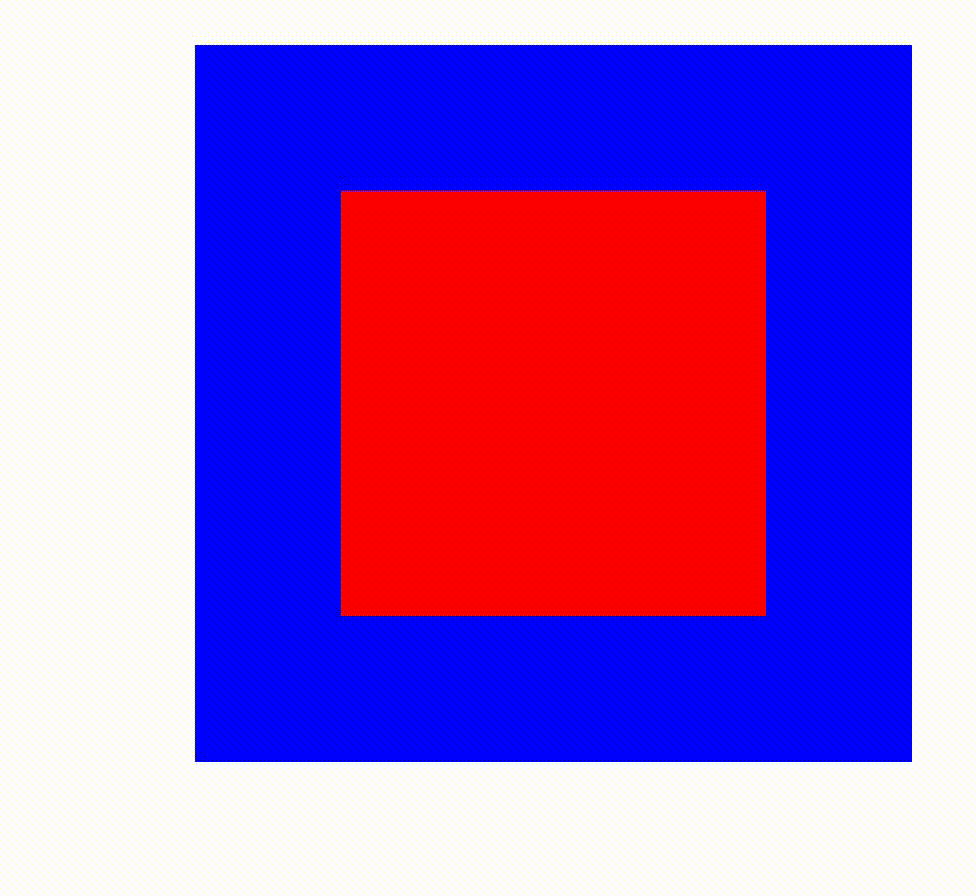For a given initial condition, i.e., $p_0(x) = p(0,x)$, mixed nonlinear diffusion problem solves a simple 2D/3D time dependent nonlinear problem:

$$\frac{\partial p}{\partial t} + \nabla\cdot \boldsymbol{v} = f\,, \qquad \nabla p = -a(p)\boldsymbol{v},$$

with a natural insulating boundary condition $\frac{\partial v}{\partial n}=0$. The $H(div)$-conforming Raviart-Thomas finite element space is used for the velocity function $\boldsymbol{v}$, and the $L^2$ finite element space is used for pressure function, $p$. This example introduces how the hyper-reduction is implemented and how the reduced bases for two field varibles, $p$ and $\boldsymbol{v}$.

One can run the following command line options to reproduce the pROM results summarized in the table below:

• offline1: mixed_nonlinear_diffusion -p 1 -offline -id 0 -sh 0.25
• offline2: mixed_nonlinear_diffusion -p 1 -offline -id 1 -sh 0.15
• merge: mixed_nonlinear_diffusion -p 1 -merge -ns 2
• reference FOM solution: mixed_nonlinear_diffusion -p 1 -offline -id 2 -sh 0.2
• online (DEIM): mixed_nonlinear_diffusion -p 1 -online -rrdim 8 -rwdim 8 -sh 0.2 -id 2
• online (S-OPT): mixed_nonlinear_diffusion -p 1 -online -rrdim 8 -rwdim 8 -sh 0.2 -id 2 -sopt
• online (EQP): mixed_nonlinear_diffusion -p 1 -online -rrdim 8 -rwdim 8 -ns 2 -sh 0.2 -id 2 -eqp -maxnnls 30
FOM solution time Hyper-reduction ROM solution time Speed-up Solution relative error
68.59 sec DEIM/S-OPT 3.6 sec 19.1 1.6e-3
EQP 0.38 sec 180.5 1.8e-3

The code that generates the numerical results above can be found in (mixed_nonlinear_diffusion.cpp). The mixed_nonlinear_diffusion.cpp is based on ex16p.cpp from MFEM and modified to support mixed finite element approach.

## DMD for linear advection with discontinuous pulses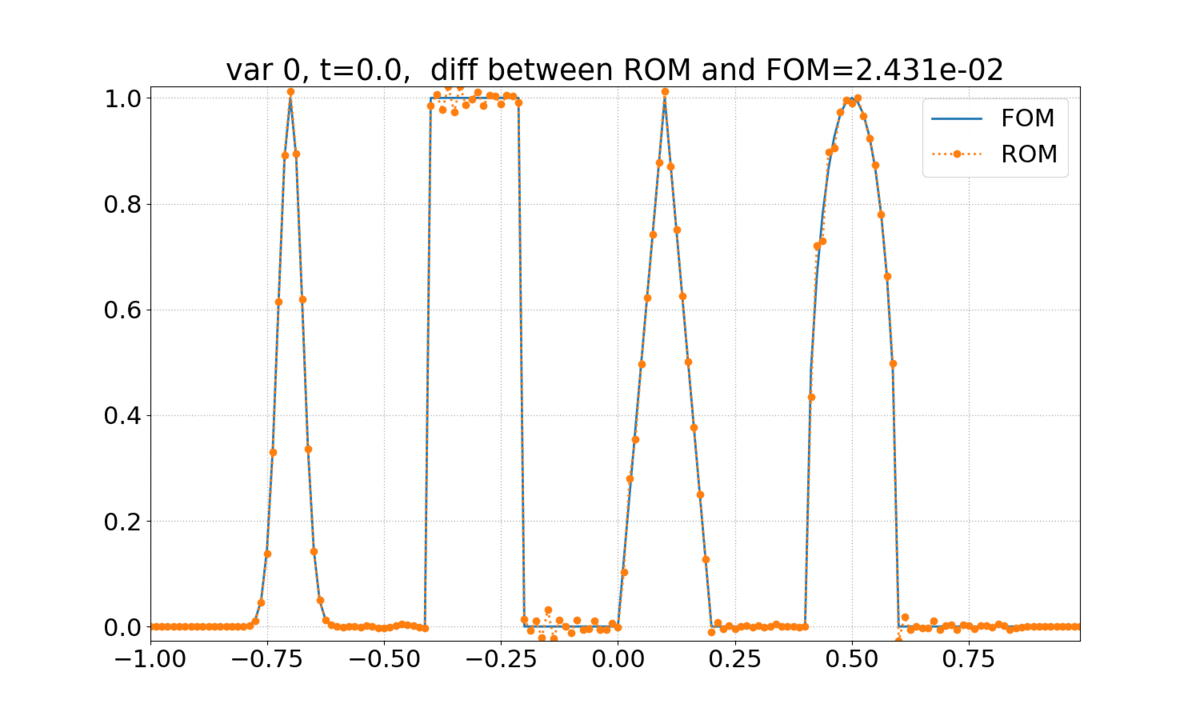For a given initial condition, i.e., $u(0,x) = u_0(x)$, 1D linear advection of the form

$$\frac{\partial u}{\partial t} + c\frac{\partial x}{\partial t} = 0,$$

where $c$ is advection velocity. The initial condition, $u_0(x)$, is given by

$$u_0(x) = \cases{ \displaystyle \exp\left (-\log(2)\frac{(x+7)^2}{0.0009}\right ) & for -0.8 \le x \le -0.6 \cr \displaystyle 1 & for -0.4 \le x \le -0.2 \cr \displaystyle 1-|10(x-0.1)| & for 0 \le x \le -0.2 \cr \displaystyle \sqrt{1-100(x-0.5)^2} & for 0.4 \le x \le 0.6 \cr \displaystyle 0 & \text{otherwise} }$$

The DMD is applied to accelerate the advection simulation:

FOM solution time DMD setup time DMD query time
3.85 sec 0.18 sec 0.027 sec

The instruction of running this simulation can be found at the HyPar page, e.g., go to Examples -> libROM Examples -> 1D Linear Advection-Discontinuous Waves.

## DMD for wave equation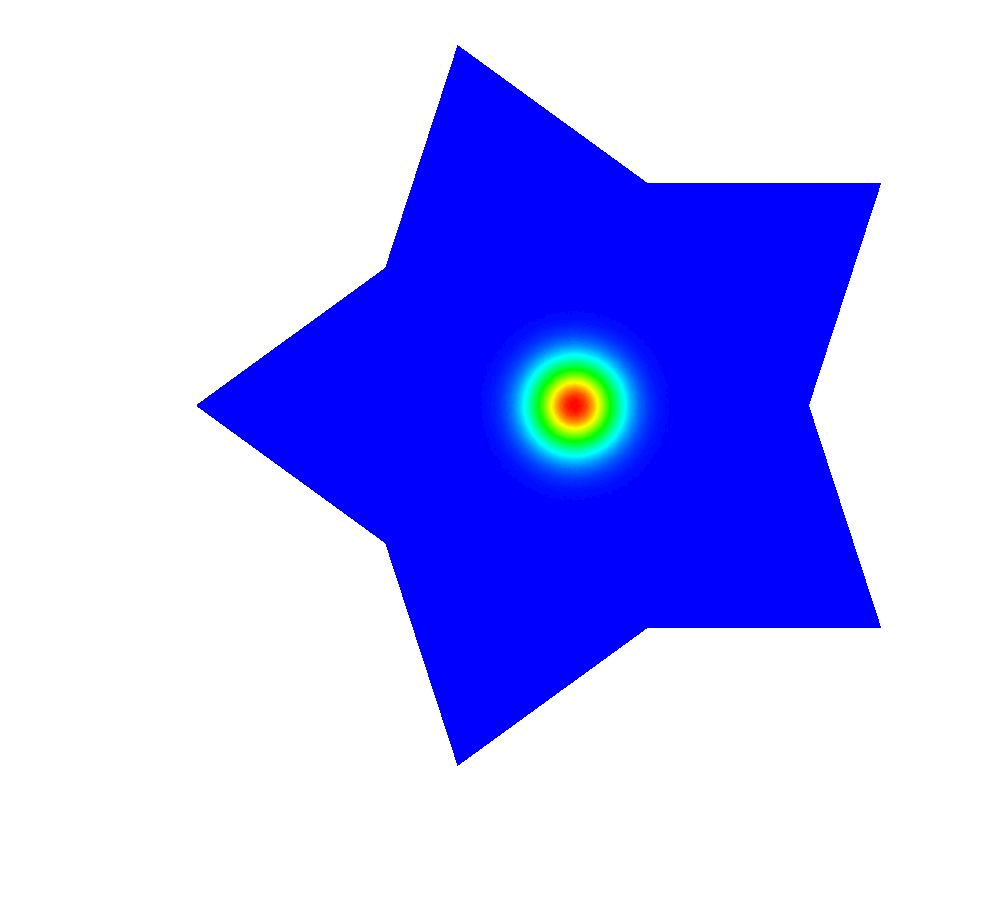For a given initial condition, i.e., $u(0,x) = u_0(x)$, and the initial rate, i.e. $\frac{\partial u}{\partial t}(0,x) = v_0(x)$, wave equation solves the time-dependent hyperbolic problem:

$$\frac{\partial^2 u}{\partial t^2} - c^2 \Delta u = 0,$$

where $c$ is a given wave speed. The boundary conditions are either Dirichlet or Neumann.

One can run the following command line options to reproduce the DMD results summarized in the table below:

• wave_equation -o 4 -tf 5 -nwinsamp 25
FOM solution time DMD setup time DMD query time DMD relative error
3.1 sec 6.9e-1 sec 2.5e-3 sec 3.0e-5

The code that generates the numerical results above can be found in (wave_equation.cpp). The wave_equation.cpp is based on ex23.cpp from MFEM.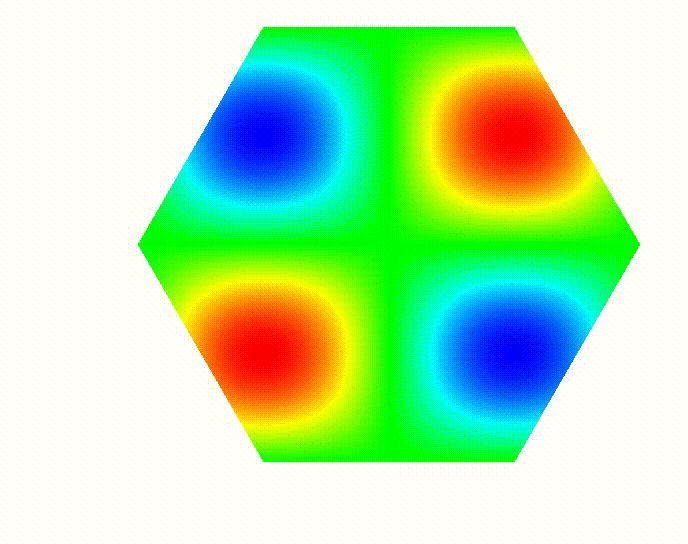For a given initial condition, i.e., $u_0(x) = u(0,x)$, DG advection solves the time-dependent advection problem:

$$\frac{\partial u}{\partial t} + v\cdot\nabla u = 0,$$

where $v$ is a given advection velocity. We choose velocity function so that the dynamics form a spiral advection.

One can run the following command line options to reproduce the DMD results summarized in the table below:

• dg_advection -p 3 -rp 1 -dt 0.005 -tf 4
FOM solution time DMD setup time DMD query time DMD relative error
5.2 sec 30.6 sec 1.9e-2 sec 1.9e-4

The code that generates the numerical results above can be found in (dg_advection.cpp). The dg_advection.cpp is based on ex9p.cpp from MFEM.For a given initial condition, i.e., $u_0(x) = u(0,x)$, DG advection solves the time-dependent advection problem:

$$\frac{\partial u}{\partial t} + v\cdot\nabla u = 0,$$

where $v$ is a given advection velocity. We choose velocity function so that the dynamics form a spiral advection.

One can run the following command line options to reproduce the pROM results summarized in the table below:

• offline1: dg_advection_global_rom -offline -ff 1.0 -id 0
• offline2: dg_advection_global_rom -offline -ff 1.1 -id 1
• offline3: dg_advection_global_rom -offline -ff 1.2 -id 2
• merge: dg_advection_global_rom -merge -ns 3
• reference FOM solution: dg_advection_global_rom -fom -ff 1.15
• online: dg_advection_global_rom -online -ff 1.15
FOM solution time pROM solution time pROM speed-up pROM relative error
1.49 sec 3.75e-3 sec 397.3 4.33e-4

The code that generates the numerical results above can be found in (dg_advection_global_rom.cpp). The dg_advection_global_rom.cpp is based on ex9p.cpp from MFEM.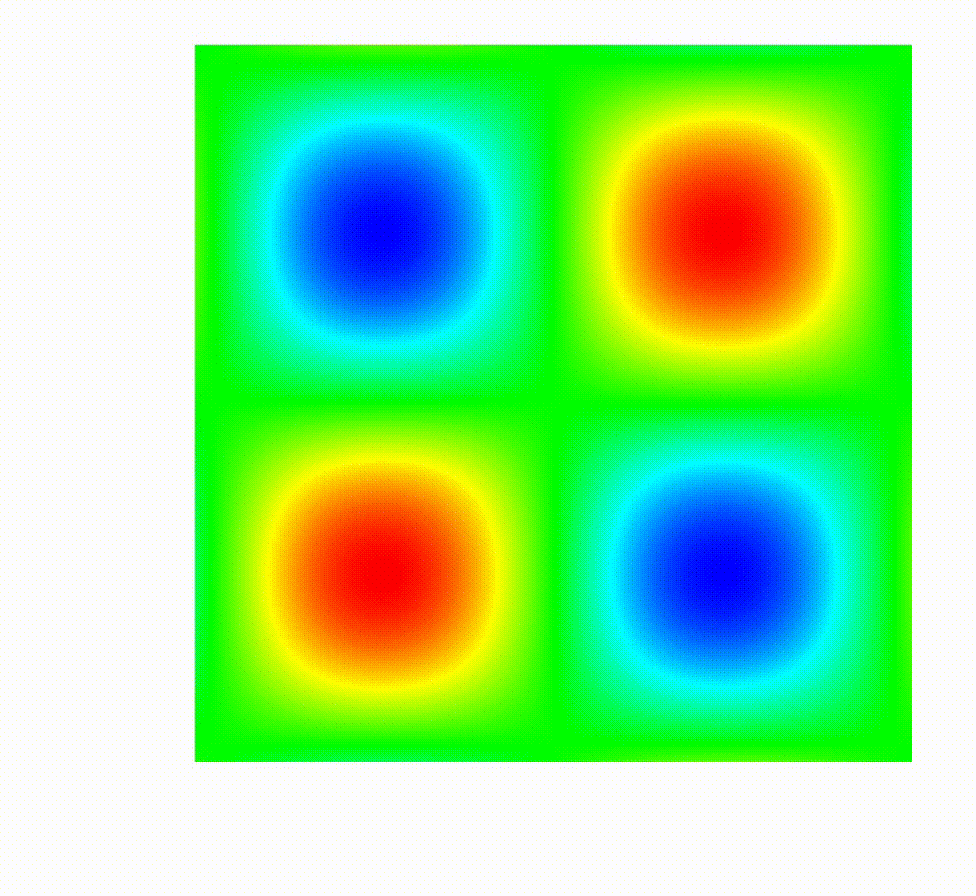For a given initial condition, i.e., $u_0(x) = u(0,x)$, DG advection solves the time-dependent advection problem:

$$\frac{\partial u}{\partial t} + v\cdot\nabla u = 0,$$

where $v$ is a given advection velocity. We choose velocity function so that the dynamics form a spiral advection.

This example illustrates how a parametric pROM can be built through local ROM interpolation techniques. The following sequence of command lines will let you build such a parametric pROM, where the frequency of sinusoidal initial condition function is used as a parameter (its value is passed by a user through -ff command line option).

Two local pROMs are constructed through -offline option with parameter values of 1.02 and 1.08, then the local pROM operators are interpolated to build a tailored local pROM at the frequency value of 1.05. Unlike the global ROM, the interpolated pROM has dimension that is the same as the individual pROM, i.e., 40 for this particular problem.

• rm -rf frequencies.txt
• dg_advection_local_rom_matrix_interp --mesh "../data/periodic-square.mesh" -offline -rs 4 -ff 1.02
• dg_advection_local_rom_matrix_interp --mesh "../data/periodic-square.mesh" -interp_prep -rs 4 -ff 1.02 -rdim 40
• dg_advection_local_rom_matrix_interp --mesh "../data/periodic-square.mesh" -offline -rs 4 -ff 1.08
• dg_advection_local_rom_matrix_interp --mesh "../data/periodic-square.mesh" -interp_prep -rs 4 -ff 1.08 -rdim 40
• dg_advection_local_rom_matrix_interp --mesh "../data/periodic-square.mesh" -fom -rs 4 -ff 1.05 -visit
• dg_advection_local_rom_matrix_interp --mesh "../data/periodic-square.mesh" -online_interp -rs 4 -ff 1.05 -rdim 40
FOM solution time pROM solution time pROM speed-up pROM relative error
39.38 sec 0.63 sec 62.5 1.19e-2

The code that generates the numerical results above can be found in (dg_advection_local_rom_matrix_interp.cpp). The dg_advection_local_rom_matrix_interp.cpp is based on ex9p.cpp from MFEM.

## DMD for sod shock tube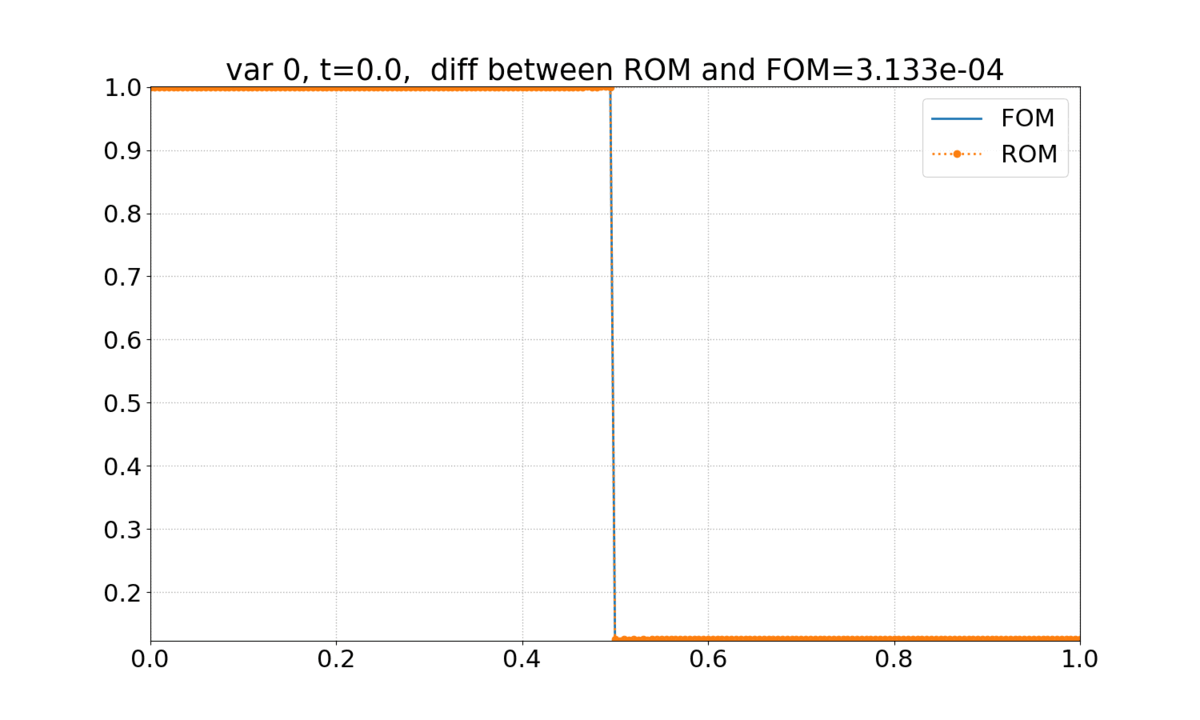1D Euler equations of the form

$$\frac{\partial \rho}{\partial t} + \frac{\partial \rho u}{\partial x} = 0$$ $$\frac{\partial \rho u}{\partial t} + \frac{\partial \rho u^2 + p}{\partial x} = 0$$ $$\frac{\partial e}{\partial t} + \frac{\partial (e+p)u}{\partial x} = 0$$

is solved with the initial condition given by

$$\rho = 1, u = 0, p = 1 \text{ for } 0 \le x < 0.5$$ $$\rho = 0.125, u = 0, p = 0.1 \text{ for } 0.5 \le x \le 1$$.

The DMD is applied to accelerate the 1D Sod shock tube simulation:

FOM solution time DMD setup time DMD query time
0.86 sec 0.13 sec 0.0027 sec

The instruction of running this simulation can be found at the HyPar page, e.g., go to Examples -> libROM Examples -> 1D Sod Shock Tube.

## DMD for isentropic vortex convection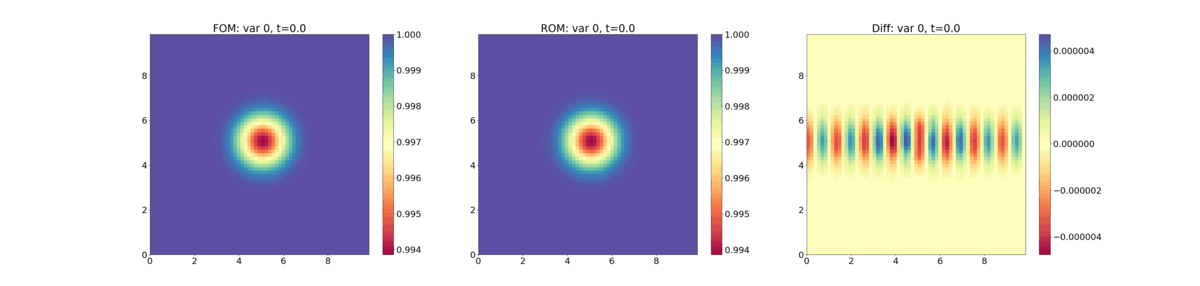2D Compressible Euler equations of the form

$$\frac{\partial \rho}{\partial t} + \frac{\partial \rho u}{\partial x} + \frac{\partial \rho v}{\partial y}= 0$$ $$\frac{\partial \rho u}{\partial t} + \frac{\partial \rho u^2 + p}{\partial x} + \frac{\partial \rho uv}{\partial y} = 0$$ $$\frac{\partial \rho v}{\partial t} + \frac{\partial \rho uv}{\partial x} + \frac{\partial \rho v^2 + p}{\partial y} = 0$$ $$\frac{\partial e}{\partial t} + \frac{\partial (e+p)u}{\partial x} + \frac{\partial (e+v)p}{\partial y} = 0$$

is solved with the free-stream condition given by

$$\rho_\infty = 1, u_\infty = 0.1, v_\infty = 0, p_\infty = 1$$

and a vortex is introduced by

$$\rho = \left ( 1-\frac{(\gamma-1)b^2}{8\gamma \pi^2} e^{1-r^2} \right )^{\frac{1}{r-1}}, p = \rho^\gamma$$ $$u = u_\infty - \frac{b}{2\pi} e^{\frac{1}{2}(1-r^2)}(y-y_c)$$ $$v = v_\infty + \frac{b}{2\pi} e^{\frac{1}{2}(1-r^2)}(x-x_c),$$

where $b=0.5$ is the vortex strength and $r = \left ( (x-x_c)^2 + (y-y_c)^2 \right )^{\frac{1}{2}}$ is the distance from the vortex center $(x_c,y_c) = (5,5)$.

The DMD is applied to accelerate the vortex convection simulation:

FOM solution time DMD setup time DMD query time
5.85 sec 5.25 sec 0.28 sec

The instruction of running this simulation can be found at the HyPar page, e.g., go to Examples -> libROM Examples -> 2D Euler Equations - Isentropic Vortex Convection.

## DMD for Riemann problem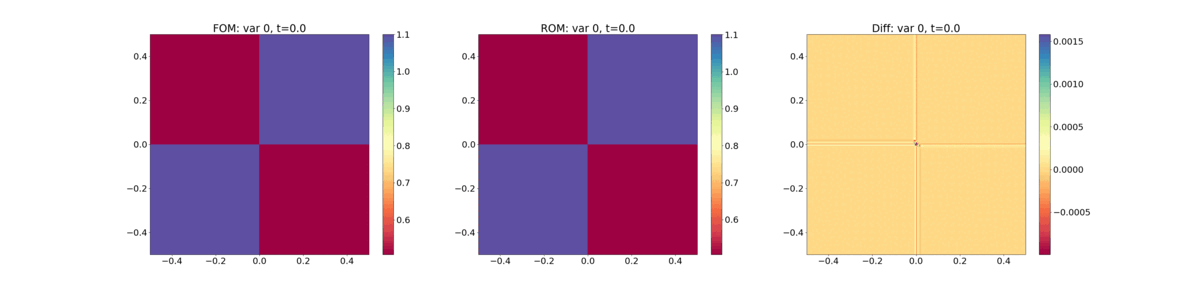2D Compressible Euler equations of the form

$$\frac{\partial \rho}{\partial t} + \frac{\partial \rho u}{\partial x} + \frac{\partial \rho v}{\partial y}= 0$$ $$\frac{\partial \rho u}{\partial t} + \frac{\partial \rho u^2 + p}{\partial x} + \frac{\partial \rho uv}{\partial y} = 0$$ $$\frac{\partial \rho v}{\partial t} + \frac{\partial \rho uv}{\partial x} + \frac{\partial \rho v^2 + p}{\partial y} = 0$$ $$\frac{\partial e}{\partial t} + \frac{\partial (e+p)u}{\partial x} + \frac{\partial (e+v)p}{\partial y} = 0$$

is solved. The DMD is applied to accelerate the Riemann problem:

FOM solution time DMD setup time DMD query time
111.1 sec 17.6 sec 1.4 sec

The instruction of running this simulation can be found at the HyPar page, e.g., go to Examples -> libROM Examples -> 2D Euler Equations - Riemann Problem Case 4

## DMD for Euler equation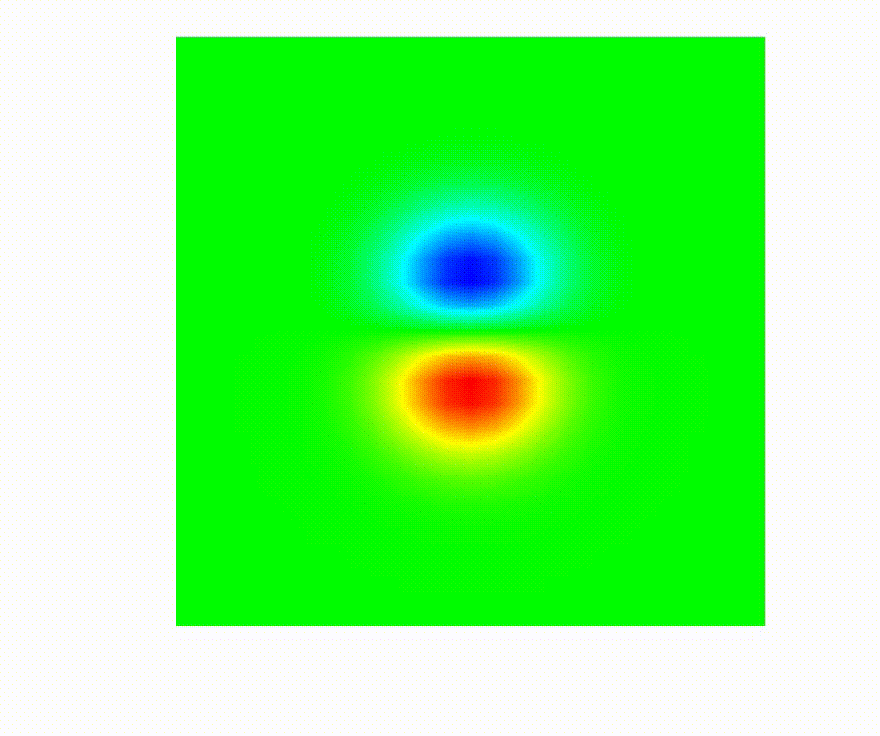For a given initial condition, i.e., $u_0(x) = u(0,x)$, DG Euler solves the compressible Euler system of equation, i.e., a model nonlinear hyperbolic PDE:

$$\frac{\partial u}{\partial t} + \nabla\cdot \boldsymbol{F}(u) = 0,$$

with a state vector $\boldsymbol{u} = [\rho,\rho v_0, \rho v_1, \rho E]$, where $\rho$ is the density, $v_i$ is the velocity in the $i$th direction, $E$ is the total specific energy, and $H = E + p/\rho$ is the total specific enthalpy. The pressure, $p$ is computed through a simple equation of state (EOS) call. The conservative hydrodynamic flux $\boldsymbol{F}$ in each direction $i$ is

$$\boldsymbol{F}_i = [\rho v_i, \rho v_0 v_i + p \delta_{i,0}, \rho v_1 v_{i,1} + p\delta_{i,1}, \rho v_i H]$$

One can run the following command line options to reproduce the DMD results summarized in the table below:

• dg_euler -p 2 -rs 2 -rp 1 -o 1 -s 3 -visit
DMD rel.error
FOM solution time DMD setup time DMD query time $\rho$ $\rho v_0$ $\rho v_1$ $E$
5.65 sec 38.9 sec 1.4e-3 sec 8.0e-7 1.2e-4 1.6e-3 2.6e-6

The code that generates the numerical results above can be found in (dg_euler.cpp). The dg_euler.cpp is based on ex18p.cpp from MFEM.

## DMD for lid-driven square cavity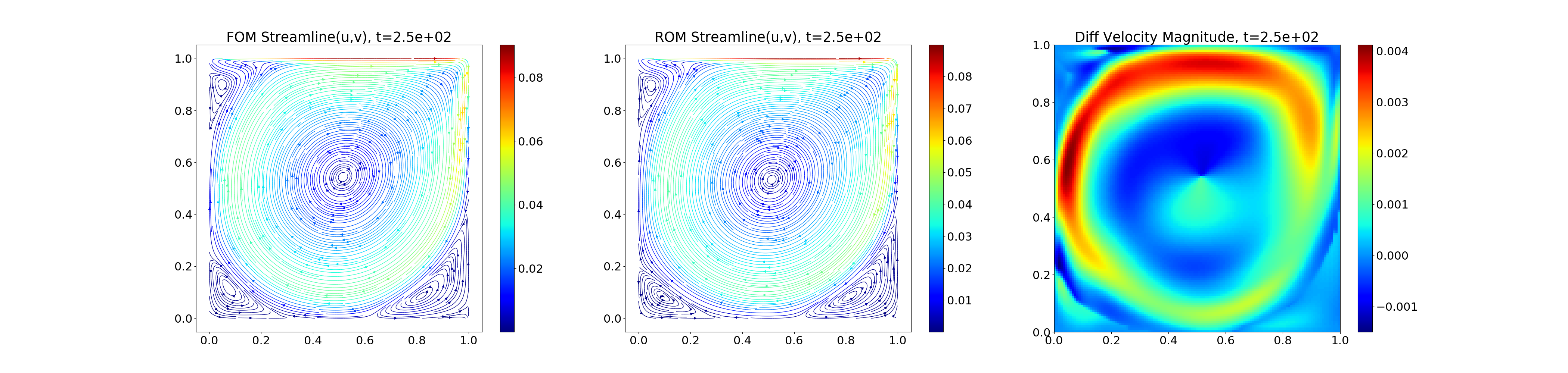A lid-driven square cavity problem is solved. The two references for this problem are

The DMD is applied to accelerate the cavity flow simulation:

FOM solution time DMD setup time DMD query time
554.6 sec 58.6 sec 0.3 sec

The instruction of running this simulation can be found at the HyPar page, e.g., go to Examples -> libROM Examples -> 2D Navier-Stokes Equations - Lid-Driven Square Cavity

## DMD for two-stream instability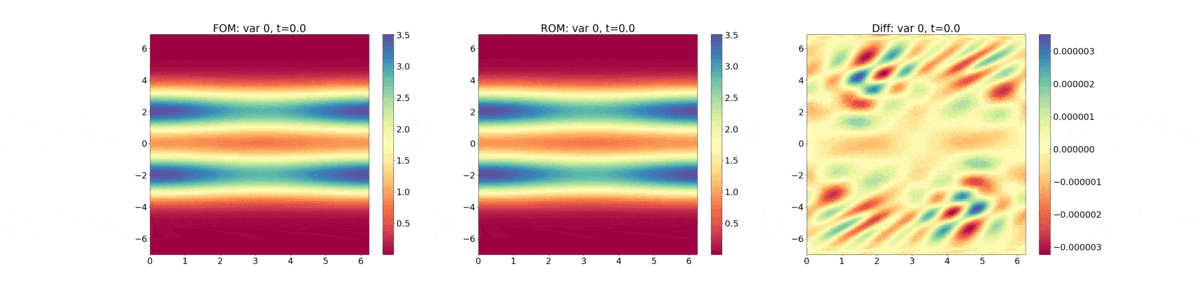The 1D-1V Vlasov equation is solved with the initial condition given by

$$f(x,v) = \frac{4}{\pi T} \left ( 1+\frac{1}{10} cos(2k\pi\frac{x}{L}) \right ) \left ( \exp\left( -\frac{(v-2)^2}{2T} \right) + \exp\left( -\frac{(v+2)^2}{2T} \right ) \right ), k=1, T=1, L=2\pi.$$

The DMD is applied to accelerate the cavity flow simulation:

FOM solution time DMD setup time DMD query time
11.34 sec 2.30 sec 0.34 sec

The instruction of running this simulation can be found at the HyPar page, e.g., go to Examples -> libROM Examples -> 2D (1D-1V) Vlasov Equation.

## Global pROM for linear elasticity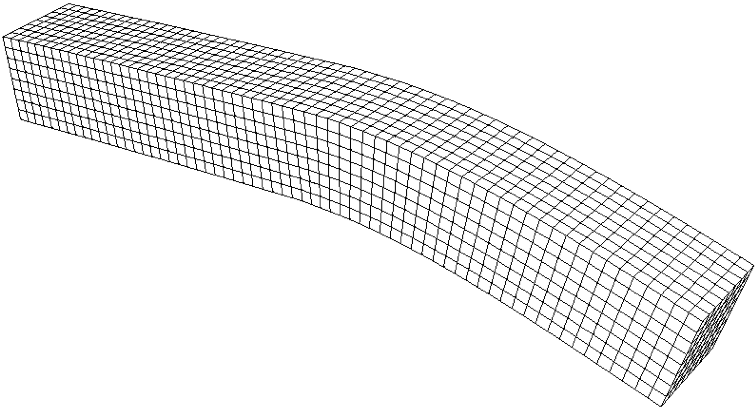This example demonstrates how to apply projection-based ROM to a linear elasticity problem. The linear elasticity problem describes a multi-material cantilever beam. Specifically, the following weak form is solved:

$$-\text{div}(\sigma(\boldsymbol{u})) = 0$$

where

$$\sigma(\boldsymbol{u}) = \lambda \text{div}(\boldsymbol{u}) \boldsymbol{I} + \mu (\nabla \boldsymbol{u} + \nabla \boldsymbol{u}^T)$$

is the stress tensor corresponding to displacement field $\boldsymbol{u}$, and $\lambda$ and $\mu$ are the material Lame constants. The Lame constants are related to Young's modulus ($E$) and Poisson's ratio ($\nu$) as

$$\lambda = \frac{E\nu}{(1+\nu)(1-2\nu)}$$ $$\mu = \frac{E}{2(1+\nu)}$$

The boundary condition are $\boldsymbol{u}=\boldsymbol{0}$ on the fixed part of the boundary with attribute 1, and $\sigma(\boldsymbol{u})\cdot n = f$ on the remainder with f being a constant pull down vector on boundary elements with attribute 2, and zero otherwise. The geometry of the domain is assumed to be as follows: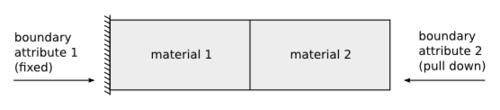Three distinct steps are required, i.e., offline, merge, and online steps, to build global ROM for the linear elasticity problem. The general description of building a global ROM is explained in this YouTube tutorial video. We parameterized Poisson's ratio ($\nu$) from 0.2 to 0.4.

One can run the following command line options to reproduce the pROM results summarized in the table below:

• offline1: linear_elasticity_global_rom --mesh "../../../dependencies/mfem/data/beam-hex-nurbs.mesh" -offline -id 0 -nu 0.2
• offline2: linear_elasticity_global_rom --mesh "../../../dependencies/mfem/data/beam-hex-nurbs.mesh" -offline -id 1 -nu 0.4
• merge: linear_elasticity_global_rom --mesh "../../../dependencies/mfem/data/beam-hex-nurbs.mesh" -merge -ns 2
• reference FOM solution: linear_elasticity_global_rom --mesh "../../../dependencies/mfem/data/beam-hex-nurbs.mesh" -offline -id 2 -nu 0.XX
• online: linear_elasticity_global_rom --mesh "../../../dependencies/mfem/data/beam-hex-nurbs.mesh" -online -id 3 -nu 0.XX

You can replace 0.XX with any value between 0.2 and 0.5. It must be strictly less than 0.5. Note that the global ROM is able to predict the point outside of the training region with high accuracy, i.e., $\nu=0.45$. The table below shows the performance results for three different parameter points.

Poisson's ratio ($\nu$) FOM solution time ROM solving time Position relative error
0.25 4.96e-2 sec 3.54e-6 sec 0.00081
0.3 4.93e-2 sec 4.37e-6 sec 0.00133
0.35 5.96e-2 sec 4.60e-6 sec 0.00121
0.45 5.22e-2 sec 4.36e-6 sec 0.00321

The code that generates the numerical results above can be found in (linear_elasticity_global_rom.cpp). The linear_elasticity_global_rom.cpp is based on ex2p.cpp from MFEM.

## Global pROM for nonlinear elasticity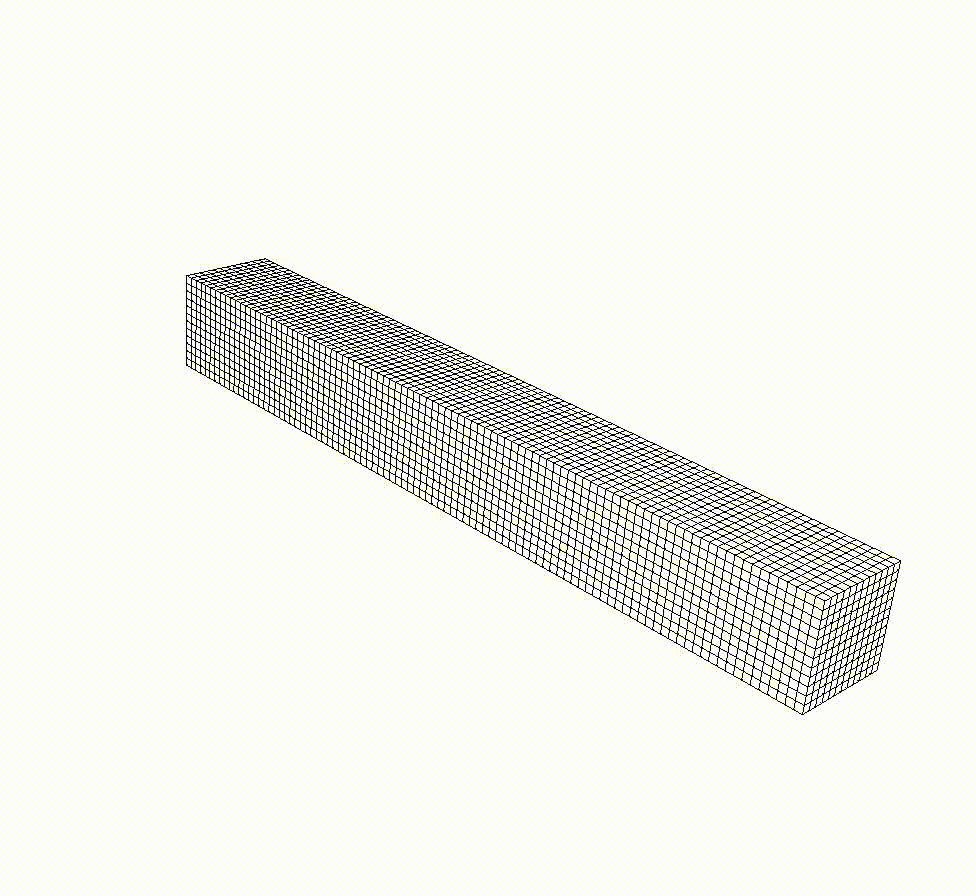For a given initial condition, i.e., $v_0(x) = v(0,x)$, nonlinear elasticity solves a time dependent nonlinear elasticity problem of the form

$$\frac{\partial v}{\partial t} = H(x) + Sv\,, \qquad \frac{\partial x}{\partial t} = v,$$

where $H$ is a hyperelastic model and $S$ is a viscosity operator of Laplacian type. The initial displacement is set zero and the initial velocity is set as zero except the third component which is defined:

$$v_3(0,x) = -\frac{\mu}{80}\sin(\mu x_1)$$

One can run the following command line options to build global ROM and reproduce the results summarizedin the table below. You can replace XXX in the fom and online phase to take any $\mu$ value between 3.9 and 4.1:

• offline1: nonlinear_elasticity_global_rom --mesh "../../../dependencies/mfem/data/beam-hex-nurbs.mesh" --offline -dt 0.01 -tf 5.0 -s 14 -vs 10 -sc 3.9 -id 0
• offline2: nonlinear_elasticity_global_rom --mesh "../../../dependencies/mfem/data/beam-hex-nurbs.mesh" --offline -dt 0.01 -tf 5.0 -s 14 -vs 10 -sc 4.1 -id 1
• merge: nonlinear_elasticity_global_rom --mesh "../../../dependencies/mfem/data/beam-hex-nurbs.mesh" --merge -ns 2 -dt 0.01 -tf 5.0
• reference FOM solution: nonlinear_elasticity_global_rom --mesh "../../../dependencies/mfem/data/beam-hex-nurbs.mesh" --offline -dt 0.01 -tf 5.0 -s 14 -vs 5 -sc XXX -id 2
• online: nonlinear_elasticity_global_rom --mesh "../../../dependencies/mfem/data/beam-hex-nurbs.mesh" --online -dt 0.01 -tf 5.0 -s 14 -vs 5 -hyp -rvdim 40 -rxdim 10 -hdim 71 -nsr 200 -sc XXX
$\mu$ FOM solution time pROM online time Speed-up Position relative error
3.92 164.9 sec 20.5 sec 8.0 0.0053
3.94 169.2 sec 20.8 sec 8.1 0.0053
3.96 167.8 sec 20.9 sec 8.0 0.0057
3.98 162.7 sec 22.1 sec 7.4 0.0062
4.0 169.4 sec 21.1 sec 8.0 0.0067
4.02 168.4 sec 20.8 sec 8.1 0.0071
4.04 160.6 sec 22.8 sec 7.0 0.0073
4.06 173.4 sec 22.7 sec 7.6 0.0071
4.08 169.2 sec 20.0 sec 8.5 0.0066

The code that generates the numerical results above can be found in (nonlinear_elasticity_global_rom.cpp). The nonlinear_elasticity_global_rom.cpp is based on ex10p.cpp from MFEM.

## DMD for nonlinear elasticityFor a given initial condition, i.e., $v_0(x) = v(0,x)$, nonlinear elasticity solves a time dependent nonlinear elasticity problem of the form

$$\frac{\partial v}{\partial t} = H(x) + Sv\,, \qquad \frac{\partial x}{\partial t} = v,$$

where $H$ is a hyperelastic model and $S$ is a viscosity operator of Laplacian type.

One can run the following command line options to reproduce the DMD results summarized in the table below:

• nonlinear_elasticity -s 2 -rs 1 -dt 0.01 -tf 5 -visit
FOM solution time DMD setup time DMD query time Position relative error Velocity relative error
10.4 sec 2.9e-1 sec 1.1 sec 7.0e-5 1.4e-3

The code that generates the numerical results above can be found in (nonlinear_elasticity.cpp). The nonlinear_elasticity.cpp is based on ex10p.cpp from MFEM.

## Global pROM for Lagrangian hydrodynamics

Laghos (LAGrangian High-Order Solver) is a miniapp that solves the time-dependent Euler equations of compressible gas dynamics in a moving Lagrangian frame using unstructured high-order finite element spatial discretization and explicit high-order time-stepping. LaghosROM introduces reduced order models of Laghos simulations.

A list of example problems that you can solve with LaghosROM includes Sedov blast, Gresho vortex, Taylor-Green vortex, triple-point, and Rayleigh-Taylor instability problems. Below are command line options for each problems and some numerical results. For each problem, four different phases need to be taken, i.e., the offline, hyper-reduction preprocessing, online, and restore phase. The online phase runs necessary full order model (FOM) to generate simulation data. libROM dynamically collects the data as the FOM simulation marches in time domain. In the hyper-reduction preprocessing phase, the libROM builds a library of reduced basis as well as hyper-reduction operators. The online phase runs the ROM and the restore phase projects the ROM solutions to the full order model dimension.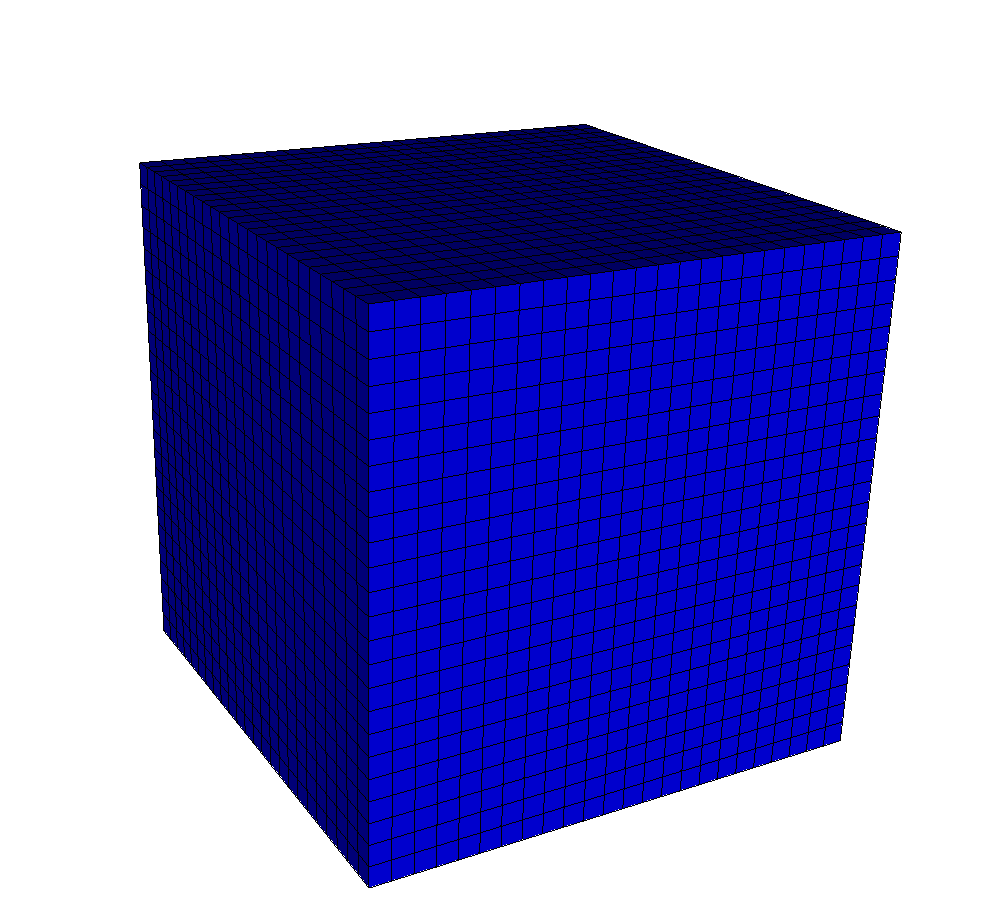### Sedov blast problem

Sedov blast problem is a three-dimensional standard shock hydrodynamic benchmark test. An initial delta source of internal energy deposited at the origin of a three-dimensional cube is considered. The computational domain is the unit cube $\tilde{\Omega} = [0,1]^3$ with wall boundary conditions on all surfaces, i.e., $v\cdot n = 0$. The initial velocity is given by $v=0$. The initial density is given by $\rho = 1$. The initial energy is given by a delta function at the origin. The adiabatic index in the ideal gas equations of state is set $\gamma = 1.4$. The initial mesh is a uniform Catesian hexahedral mesh, which deforms over time. It can be seen that the radial symmetry is maintained in the shock wave propagation in both FOM and pROM simulations. One can reproduce the pROM numerical result, following the command line options described below:

• offline: laghos -o twp_sedov -m ../data/cube01_hex.mesh -pt 211 -tf 0.8 -s 7 -pa -offline -visit -romsvds -ef 0.9999 -writesol -romos -rostype load -romsns -nwinsamp 21 -sample-stages
• hyper-reduction preprocessing: laghos -o twp_sedov -m ../data/cube01_hex.mesh -pt 211 -tf 0.8 -s 7 -pa -online -romsvds -romos -rostype load -romhrprep -romsns -romgs -nwin 66 -sfacv 2 -sface 2 (-sopt)
• online: laghos -o twp_sedov -m ../data/cube01_hex.mesh -pt 211 -tf 0.8 -s 7 -pa -online -romsvds -romos -rostype load -romhr -romsns -romgs -nwin 66 -sfacv 2 -sface 2
• restore: laghos -o twp_sedov -m ../data/cube01_hex.mesh -pt 211 -tf 0.8 -s 7 -pa -restore -soldiff -romsvds -romos -rostype load -romsns -romgs -nwin 66
FOM solution time ROM solution time Speed-up Velocity relative error (DEIM) Velocity relative error (SOPT)
191 sec 8.3 sec 22.8 2.2e-4 1.1e-4

One can also easily apply time-windowing DMD to Sedov blast problem easily. First, prepare tw_sedov3.csv file, which contains a sequence of time steps, {0.01, 0.02, $\ldots$, 0.79, 0.8 } in a column. Then you can follow the command line options described below:

• offline: laghos -o dmd_sedov -p 4 -m ../data/cube01_hex.mesh -pt 211 -tf 0.8 -s 7 -pa -offline -visit -romsvds -ef 0.9999 -writesol -nwin 80 -tw tw_sedov3.csv -dmd -dmdnuf -met -no-romoffset

• online: laghos -o dmd_sedov -p 4 -m ../data/cube01_hex.mesh -pt 211 -tf 0.8 -s 7 -pa -restore -soldiff -romsvds -dmd -dmdnuf -no-romoffset

FOM solution time DMD restoration time Speed-up Velocity relative error
30.4 sec 15.0. sec 2.0 0.0382461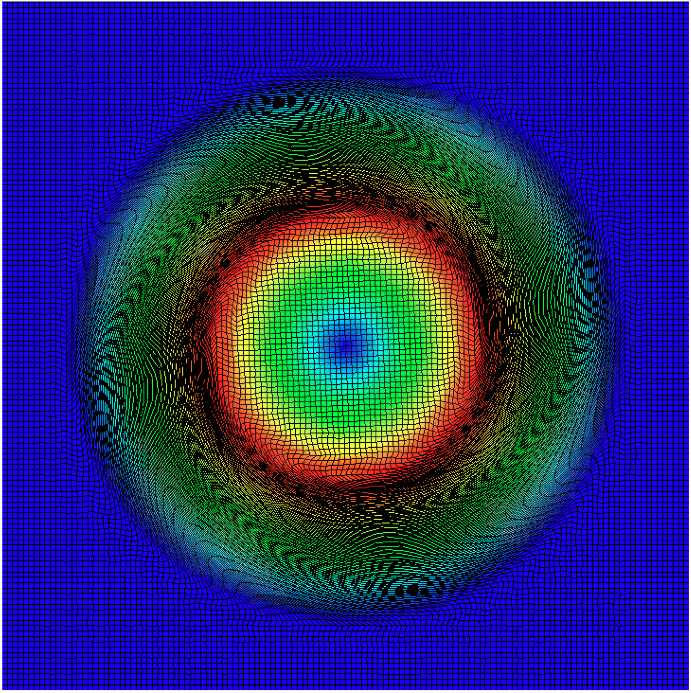### Gresho vortex problem

Gresho vortex problem is a two-dimensional benchmark test for the incompressible inviscid Navier-Stokes equations. The computational domain is the unit square $\tilde\Omega = [-0.5,0.5]^2$ with wall boundary conditions on all surfaces, i.e., $v\dot n = 0$. Let $(r,\phi)$ denote the polar coordinates of a particle $\tilde{x} \in \tilde{\Omega}$. The initial angular velocity is given by

$$v_\phi = \cases{ \displaystyle 5r & for 0 \leq r < 0.2 \cr \displaystyle 2-5r & for 0.2 \leq r < 0.4 \cr \displaystyle 0 i & for r \geq 0.4. }$$

The initial density if given by $\rho=1$. The initial thermodynamic pressure is given by

$$p = \cases{ 5 + \frac{25}{2} r^2 & for 0 \leq r < 0.2 \cr 9 - 4 \log(0.2) + \frac{25}{2} - 20r + 4 \log(r) & for 0.2 \leq r < 0.4 \cr 3 + 4\log(2) & for r \geq 0.4 }$$

• offline: laghos -o twp_gresho -p 4 -m ../data/square_gresho.mesh -rs 4 -ok 3 -ot 2 -tf 0.62 -s 7 -visit -writesol -offline -ef 0.9999 -romsvds -romos -rostype load -romsns -nwinsamp 21 -sample-stages
• hyper-reduction preprocessing: laghos -o twp_gresho -p 4 -m ../data/square_gresho.mesh -rs 4 -ok 3 -ot 2 -tf 0.62 -s 7 -online -romhrprep -romsvds -romos -rostype load -romsns -romgs -nwin 152 -sfacv 2 -sface 2
• online: laghos -o twp_gresho -p 4 -m ../data/square_gresho.mesh -rs 4 -ok 3 -ot 2 -tf 0.62 -s 7 -online -romhr -romsvds -romos -rostype load -romsns -romgs -nwin 152 -sfacv 2 -sface 2
• restore: laghos -o twp_gresho -p 4 -m ../data/square_gresho.mesh -rs 4 -ok 3 -ot 2 -tf 0.62 -s 7 -soldiff -restore -romsvds -romos -rostype load -romsns -romgs -nwin 152
FOM solution time ROM solution time Speed-up Velocity relative error
218 sec 8.4 sec 25.9 2.1e-4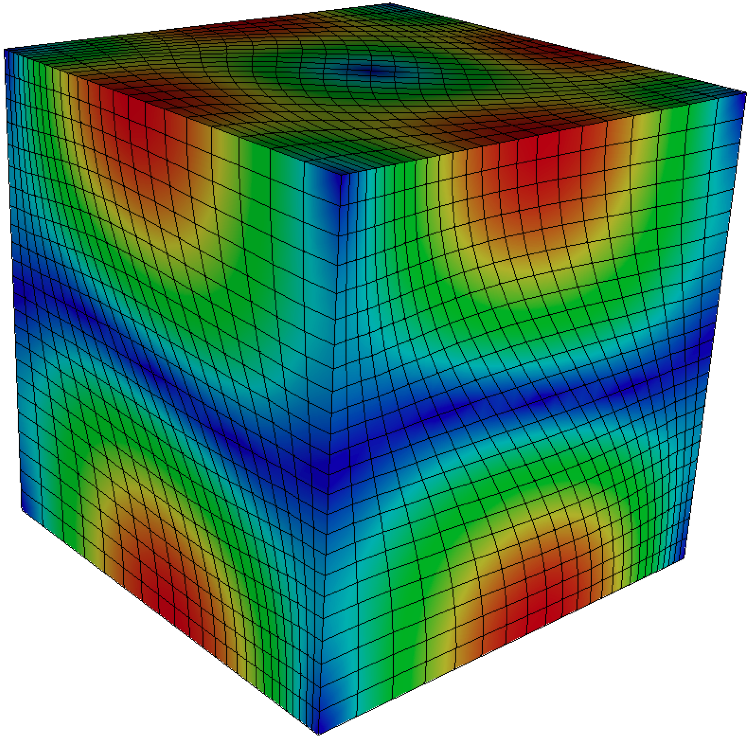### Taylor-Green vortex

Taylor-Green vortex problem is a three-dimensional benchmark test for the incompressible Navier-Stokes equasions. A manufactured smooth solution is considered by extending the steady state Taylor-Green vortex solution to the compressible Euler equations. The computational domain is the unit cube $\tilde{\Omega}=[0,1]^3$ with wall boundary conditions on all surfaces, i.e., $v\cdot n = 0$. The initial velocity is given by

$$v = (\sin{(\pi x)} \cos{(\pi y)} \cos{(\pi z)}, -\cos{(\pi x)}\sin{(\pi y)}\cos{(\pi z)}, 0)$$

The initial density is given by $\rho =1$. The initial thermodynamic pressure is given by

$$p = 100 + \frac{(\cos{(2\pi x)} + \cos{(2\pi y))(\cos{(2\pi z)+2})-2}}{16}$$

The initial energy is related to the pressure and the density by the equation of state for the ideal gas, $p=(\gamma-1)\rho e$, with $\gamma = 5/3$. The initial mesh is a uniform Cartesian hexahedral mesh, which deforms over time. The visualized solution is given on the right. One can reproduce the numerical result, following the command line options described below:

• offline: laghos -o twp_taylor -m ../data/cube01_hex.mesh -p 0 -rs 2 -cfl 0.1 -tf 0.25 -s 7 -pa -offline -visit -romsvds -ef 0.9999 -writesol -romos -rostype load -romsns -nwinsamp 21 -sdim 1000 -sample-stages
• hyper-reduction preprocessing: laghos -o twp_taylor -m ../data/cube01_hex.mesh -p 0 -rs 2 -cfl 0.1 -tf 0.25 -s 7 -pa -online -romsvds -romos -rostype load -romhrprep -romsns -romgs -nwin 82 -sfacv 2 -sface 2
• online: laghos -o twp_taylor -m ../data/cube01_hex.mesh -p 0 -rs 2 -cfl 0.1 -tf 0.25 -s 7 -pa -online -romsvds -romos -rostype load -romhr -romsns -romgs -nwin 82 -sfacv 2 -sface 2
• restore: laghos -o twp_taylor -m ../data/cube01_hex.mesh -p 0 -rs 2 -cfl 0.1 -tf 0.25 -s 7 -pa -restore -soldiff -romsvds -romos -rostype load -romsns -romgs -nwin 82
FOM solution time ROM solution time Speed-up Velocity relative error
170 sec 5.4 sec 31.2 1.1e-6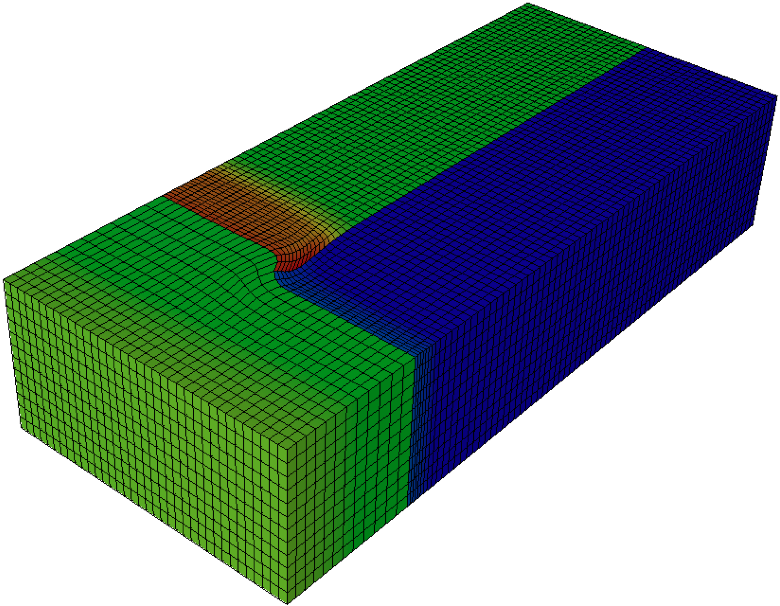### Triple-point problem

Triple-point problem is a three-dimensional shock test with two materials in three states. The computational domain is $\tilde{\Omega} = [0,7] \times [0,3 ] \times [0,1.5]$ with wall boundary conditions on all surfaces, i.e., $v\cdot n = 0$. The initial velocity is given by $v=0$. The initial density is given by

$$\rho = \cases{ \displaystyle 1 & for x \leq 1 or y \leq 1.5, \cr \displaystyle 1/8 & for x > 1 and y > 1.5 }$$

The initial thermodynamic pressure is given for

$$p = \cases{ \displaystyle 1 & for x \leq 1, \cr \displaystyle 0.1 & for x > 1 }$$

The initial energy is related to the pressure and the density by the equation of state for the ideal gas, $p=(\gamma-1)\rho e$, with

$$\gamma = \cases{ \displaystyle 1.5 & for x \leq 1 or y > 1.5\cr \displaystyle 1.4 & for x > 1 and y \leq 1.5 }$$

The initial mesh is a uniform Cartesian hexahedral mesh, which deforms over time. The visualized solution is given on the right. One can reproduce the numerical result, following the command line options described below:

• offline: laghos -o twp_triple -p 3 -m ../data/box01_hex.mesh -rs 2 -tf 0.8 -s 7 -cfl 0.5 -pa -offline -writesol -visit -romsvds -romos -rostype load -romsns -nwinsamp 21 -ef 0.9999 -sdim 200 -sample-stages
• hyper-reduction preprocessing: laghos -o twp_triple -p 3 -m ../data/box01_hex.mesh -rs 2 -tf 0.8 -s 7 -cfl 0.5 -pa -online -romhrprep -romsvds -romos -rostype load -romgs -romsns -nwin 18 -sfacv 2 -sface 2
• online: laghos -o twp_triple -p 3 -m ../data/box01_hex.mesh -rs 2 -tf 0.8 -s 7 -cfl 0.5 -pa -online -romhr -romsvds -romos -rostype load -romgs -romsns -nwin 18 -sfacv 2 -sface 2
• restore: laghos -o twp_triple -p 3 -m ../data/box01_hex.mesh -rs 2 -tf 0.8 -s 7 -cfl 0.5 -pa -restore -soldiff -romsvds -romos -rostype load -romgs -romsns -nwin 18
FOM solution time ROM solution time Speed-up Velocity relative error
122 sec 1.4 sec 87.8 8.1e-4### Rayleigh-Taylor instability problem

Rayleigh-Taylor instability problem

• offline: laghos -p 7 -m ../data/rt2D.mesh -tf 1.5 -rs 4 -ok 2 -ot 1 -pa -o twp_rt -s 7 -writesol -offline -romsns -sdim 200000 -romsvds -romos -romgs -nwinsamp 21 -ef 0.9999999999 -sample-stages
• hyper-reduction preprocessing: laghos -p 7 -m ../data/rt2D.mesh -tf 1.5 -rs 4 -ok 2 -ot 1 -pa -o twp_rt -s 7 -online -romsns -romos -romgs -nwin 187 -sfacv 2 -sface 2 -romhrprep
• online: laghos -p 7 -m ../data/rt2D.mesh -tf 1.5 -rs 4 -ok 2 -ot 1 -pa -o twp_rt -s 7 -online -romsns -romos -romgs -nwin 187 -sfacv 2 -sface 2 -romhr
• restore: laghos -p 7 -m ../data/rt2D.mesh -tf 1.5 -rs 4 -ok 2 -ot 1 -pa -o twp_rt -s 7 -restore -romsns -romos -romgs -soldiff -nwin 187
FOM solution time ROM solution time Speed-up Velocity relative error
127 sec 8.7 sec 14.6 7.8e-3

LaghosROM is an external miniapp, available at https://github.com/CEED/Laghos/tree/rom/rom.

No examples or miniapps match your criteria.